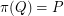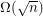# Extension complexity of (convex) polygons

 Importance: Medium ✭✭
 Author(s):
 Subject: Geometry » Polytopes
 Keywords: polytope, projection, extension complexity, convex polygon
 Posted by: DOT on: August 13th, 2011

The extension complexity of a polytopeis the minimum numberfor which there exists a polytopewithfacets and an affine mappingwith.

Question   Does there exists, for infinitely many integers, a convex polygon onvertices whose extension complexity is?

The extension complexity of a polytope is bounded from above by its number of vertices. Thus, a convex polygon withvertices has extension complexity.

Some regular convex polygons have extension complexity[BTN].

A convex polygon whose points are drawn randomly on a circle has extension complexitywith probability one (follows from [FRT]).

The question asks for the maximal extension complexity of a convex polygon.

A strongly related question is the following.

Question   What is the extension complexity of an-vertex convex polygon whose vertices are drawn randomly on a circle?

## Bibliography

*[BTN] Ben-Tal, A and Nemirovski, A. On polyhedral approximations of the second-order cone. Math. Oper. Res. 26:2 193-205 (2001)

[FRT] Fiorini, S. and Rothvoss, T. and Tiwary, H.R. Extended formulations of polygons. arXiv:1107.0371

* indicates original appearance(s) of problem.